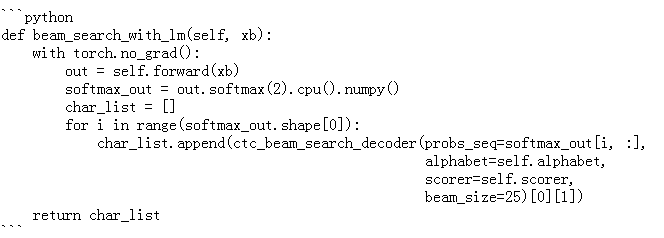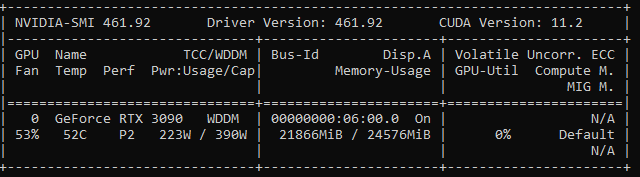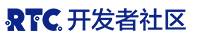理论

Nvidia对 CTC ASR 管道架构的总结

python
import librosa
import librosa.display as display
import torch
import torchaudio
import matplotlib.pyplot as plt
import numpy as np

waveform, sample_rate,  _, _, _, _ = train_dataset
num_channels, num_frames = waveform.shape
audio_transforms = torchaudio.transforms.MelSpectrogram()
spectrograms = audio_transforms(waveform)
img = spectrograms.log2().permute(1, 2, 0)
f, (ax, ax1, ax2) = plt.subplots(3, 1)
f.set_size_inches(15, 8)
ax.title.set_text('Waveform')
ax.grid(True)
ax.plot(torch.arange(0, num_frames) / sample_rate, waveform, linewidth=1)
ax1.title.set_text('Mel Spectrogram')
ax1.imshow(img)

spec = np.abs(librosa.stft(waveform.numpy().squeeze(0), hop_length=512))
spec = librosa.amplitude_to_db(spec, ref=np.max)
display.specshow(spec, sr=sample_rate, x_axis='time', y_axis='log')
plt.colorbar(format='%+2.0f dB')
ax2.title.set_text('Spectrogram')
plt.show()

• 频谱图是一幅图像，因此我们可以使用 卷积层 从中提取特征。在这篇文章中，我将使用的当红组合 Conv2d 层和 GELU 激活功能（因为 RELU 更好）与 dropout 的正规化建设。此外，我认为使用 层归一化 跳过连接 以获得更快的收敛和更好的泛化是有益的。因此，神经网络的第一部分将由以下层组成：
# First Conv2d layer</strong>
Conv2d(1, 32, kernel_size=(3, 3), stride=(2, 2), padding=(1, 1)) <strong># 7 blocks of these layers
# Skip connection is added to this Conv2d layer</strong>
LayerNorm((64,), eps=1e-05, elementwise_affine=True)
GELU()
Dropout(p=0.2, inplace=False)
Conv2d(32, 32, kernel_size=(3, 3), stride=(1, 1), padding=(1, 1))
• 同时，频谱图是时间序列数据，因此使用 GRU 等双向 RNN 层从 CNN 层检测到的特征中捕获时频模式是很自然的。出于与之前相同的原因，我将使用层规范化和 dropout：
**# I will use 5 blocks of these layers**
LayerNorm((512,), eps=1e-05, elementwise_affine=True)
GELU()
GRU(512, 512, batch_first=True, bidirectional=True)
Dropout(p=0.2, inplace=False)
• 实际上，我们需要的是将频谱图图像的每个小的垂直切片映射到某个字符，因此我们的模型将为每个垂直特征向量和每个字符生成概率分布。可以使用 线性 （全连接）层来完成：
<strong># Input for this layer is an output from the last GRU layer
# We need to gradually reduce number of outputs from 1024 to the number of characters used in LibriSpeech dataset - 28 + 'blank'</strong>
Linear(in_features= **1024** , out_features= **512** , bias=True)
GELU()
Dropout(p=0.2, inplace=False)
Linear(in_features= **512** , out_features= **29** , bias=True)
• 然后我们可以使用贪心解码器或波束搜索解码器来生成最终转录。

Speech recognition model’s architecture (1,645,181 trainable parameters)

实践

数据集

```python
target_dir = "./data"
if not os.path.isdir(target_dir):
os.makedirs(target_dir)
classes = "' abcdefghijklmnopqrstuvwxyz"

audio_transforms = torchaudio.transforms.MelSpectrogram()
num_to_char_map = {c: i for i, c in enumerate(list(classes))}
char_to_num_map = {v: k for k, v in num_to_char_map.items()}
str_to_num = lambda text: [num_to_char_map[c] for c in text]
num_to_str = lambda labels: ''.join([char_to_num_map[i] for i in labels])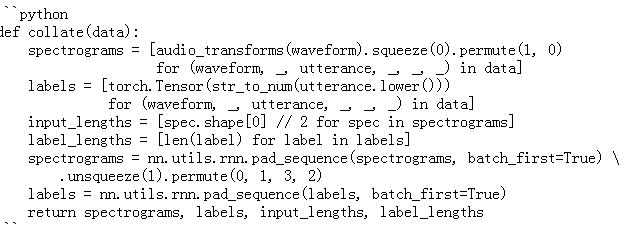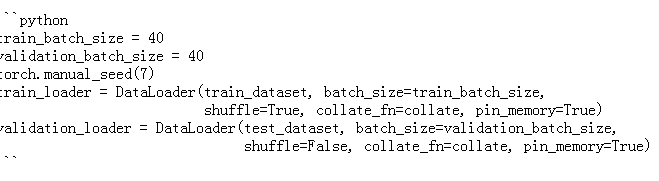训练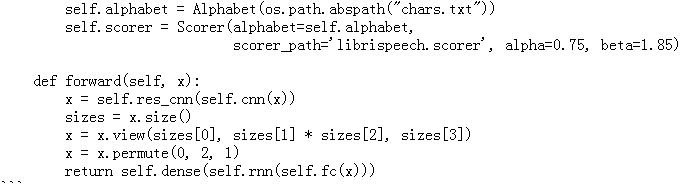text_file = open("chars.txt", "w", encoding='utf-8')
text_file.write('\n'.join(list(classes)))
text_file.close()

KenLM 模型和外部 .scorer 生成